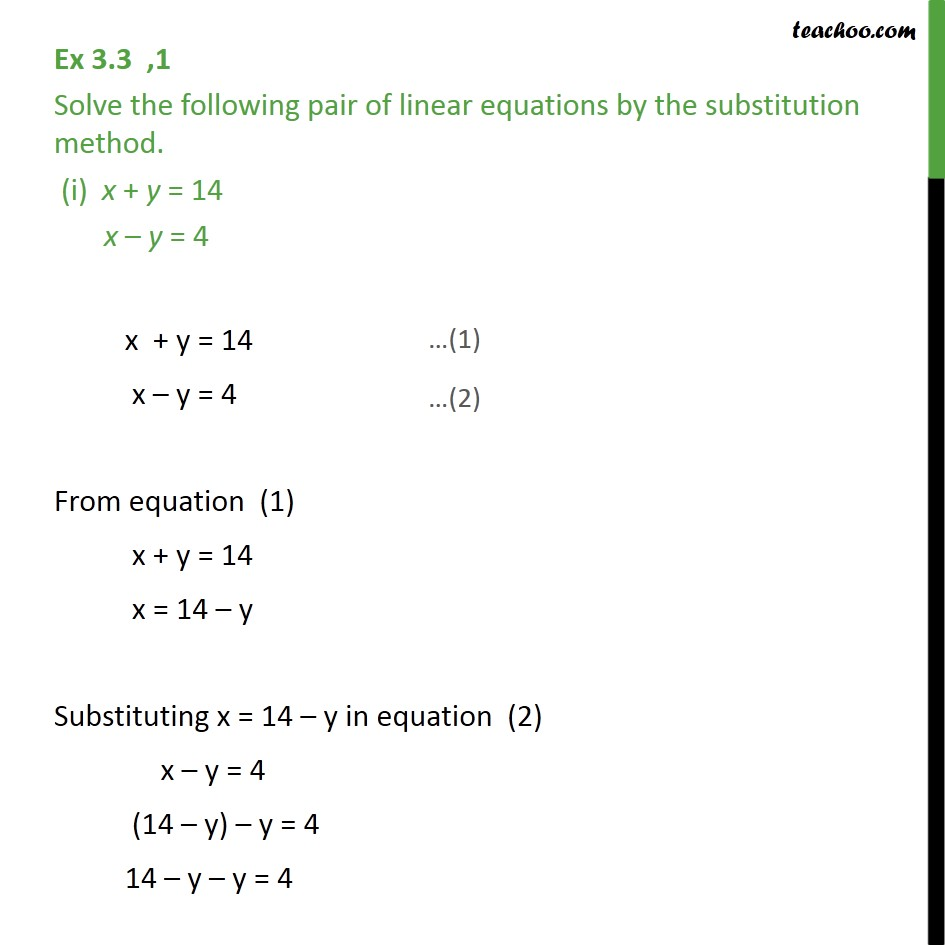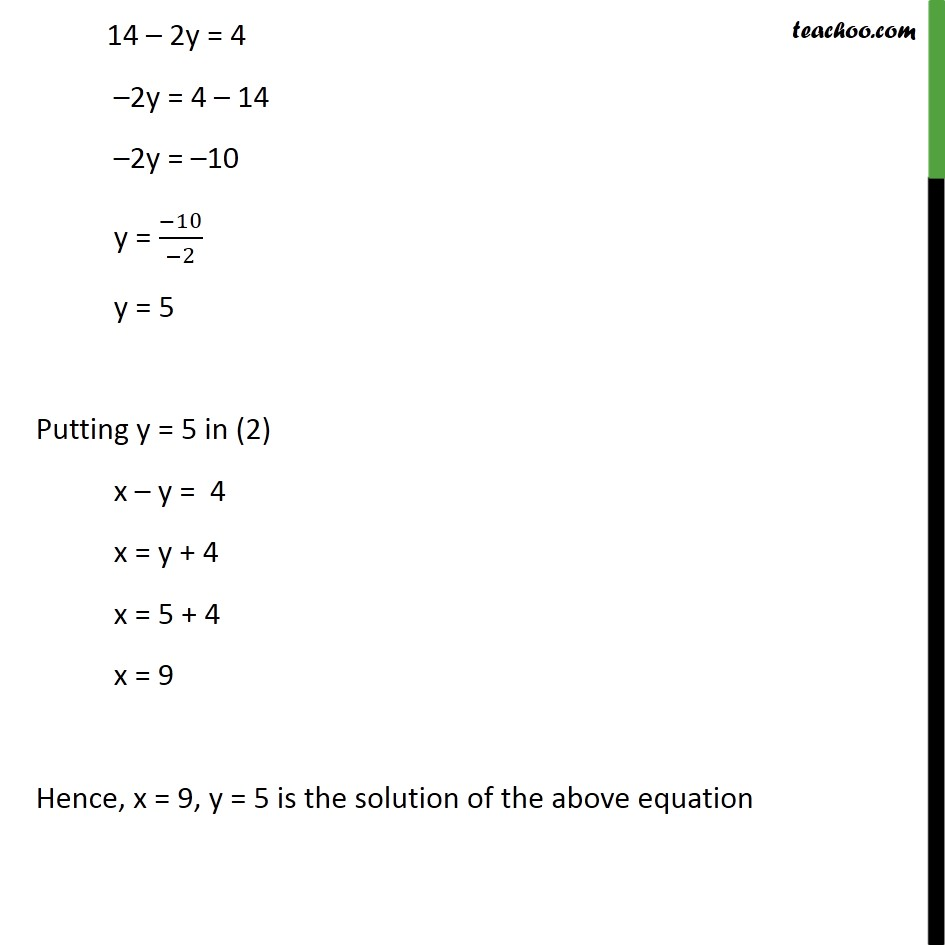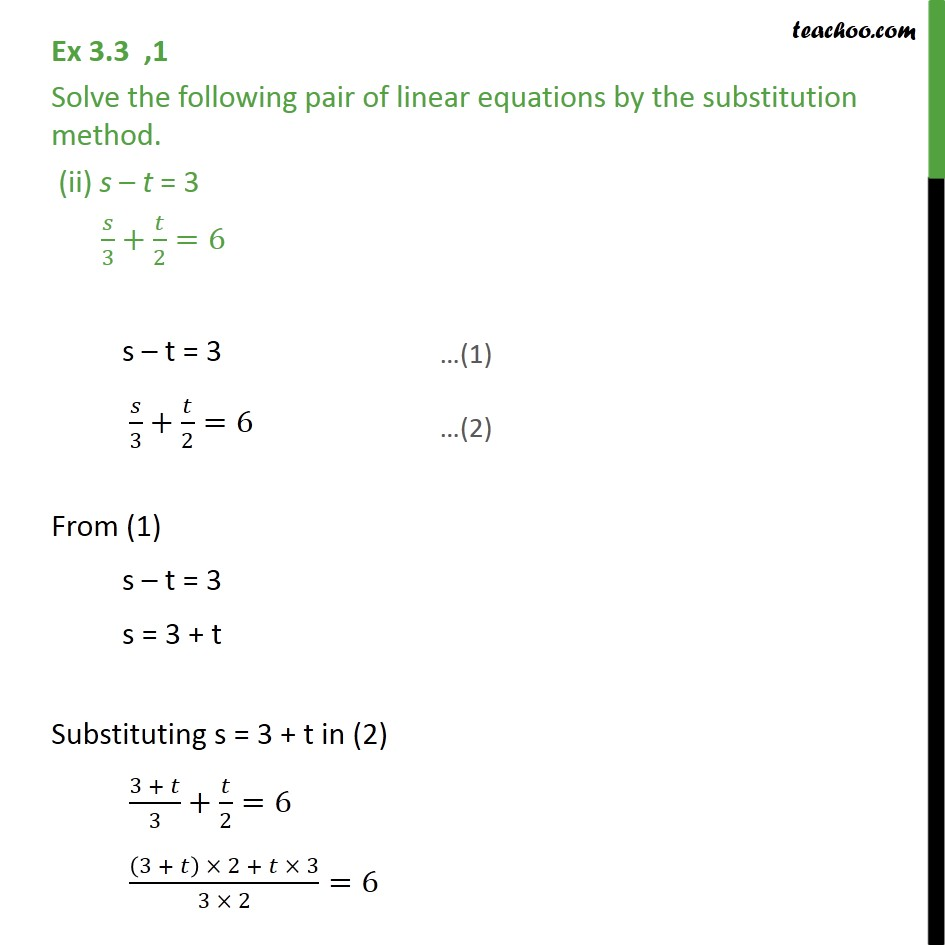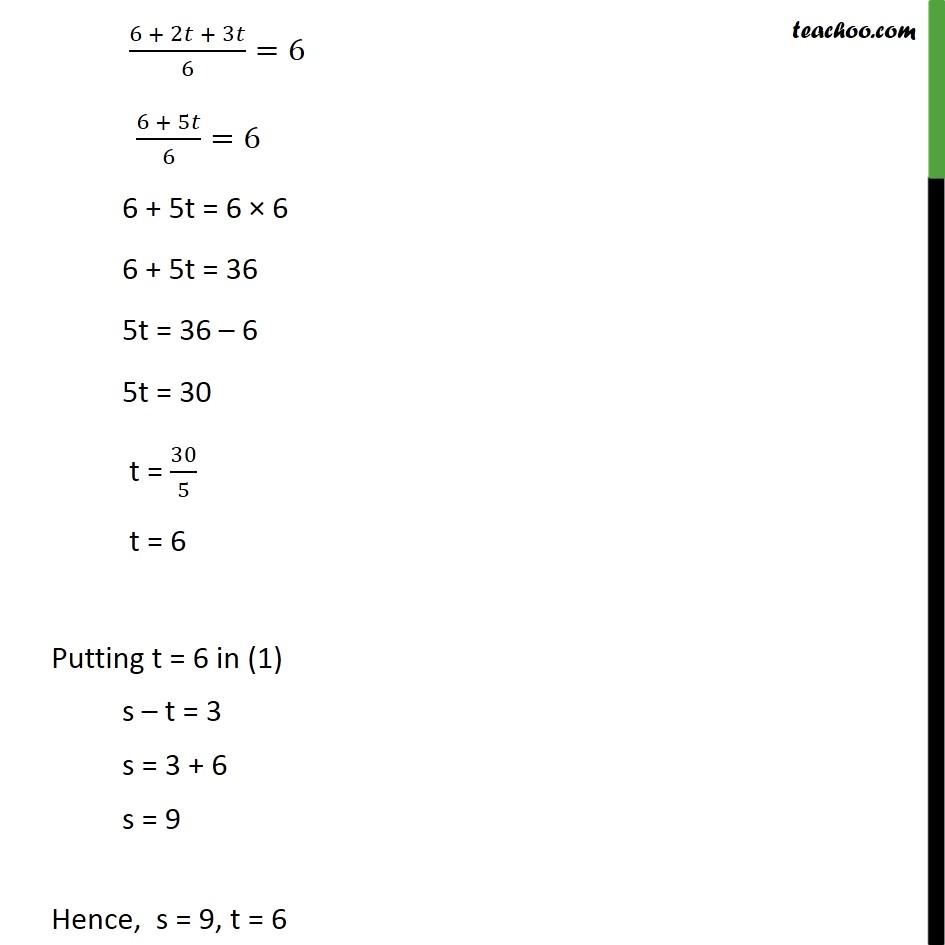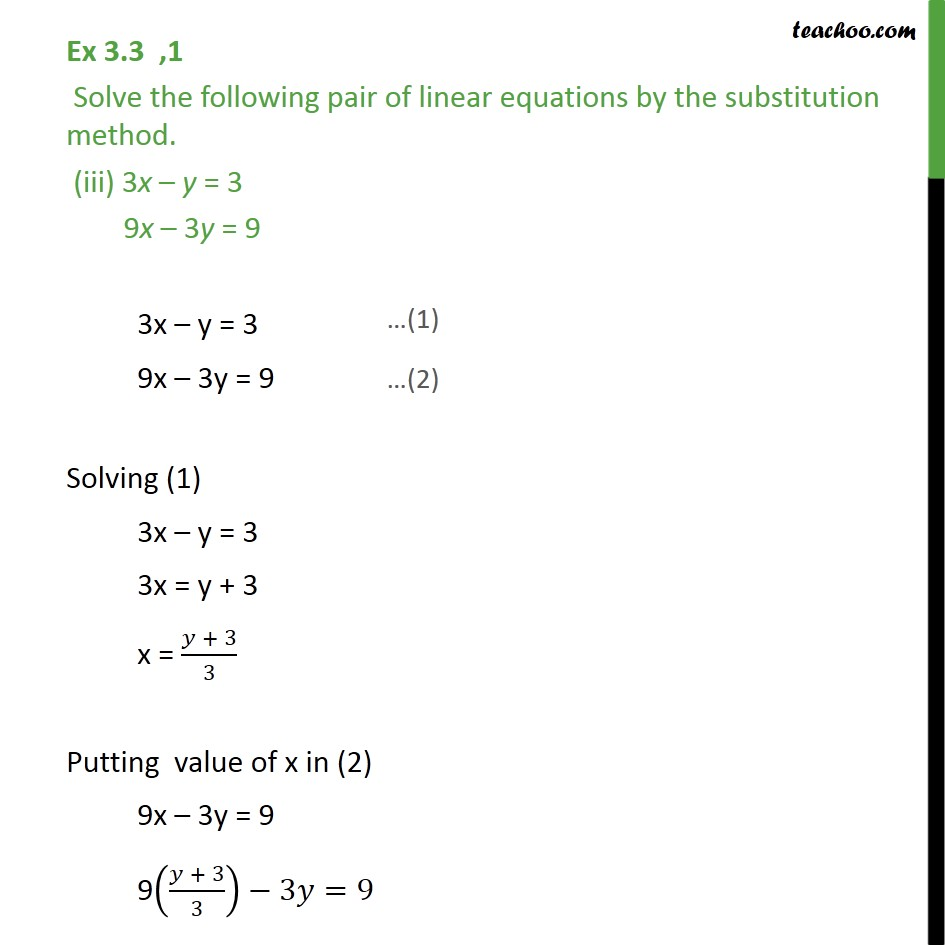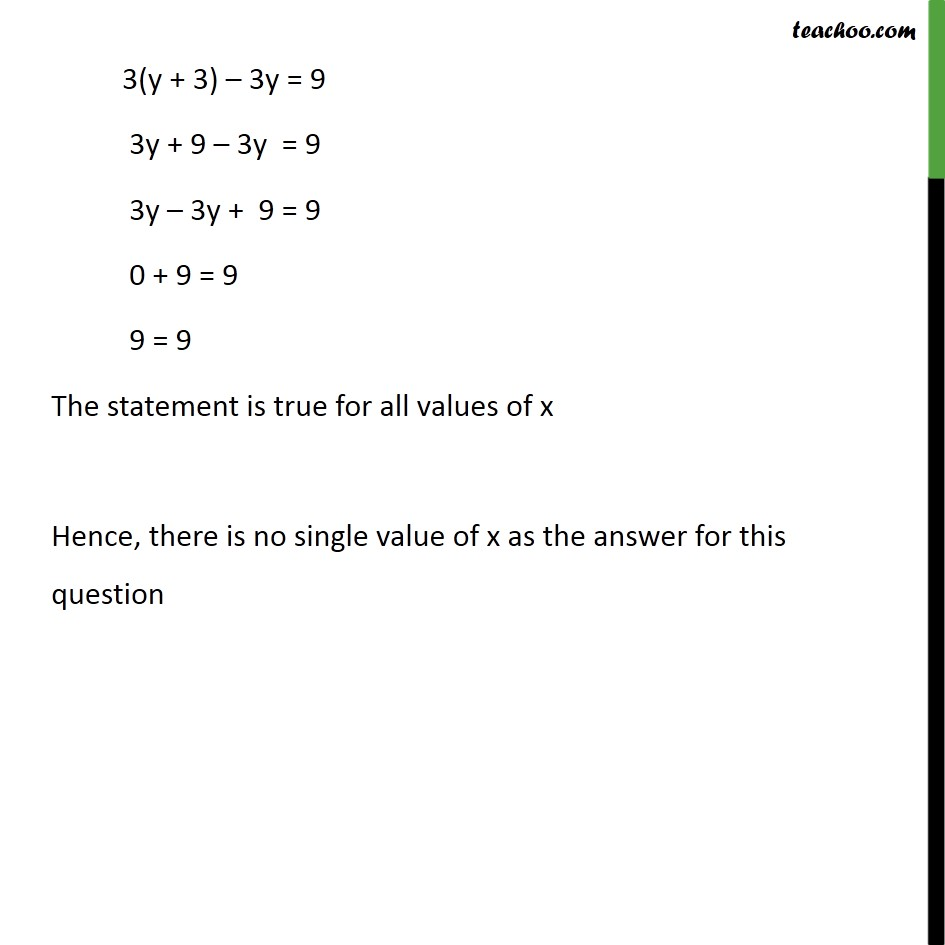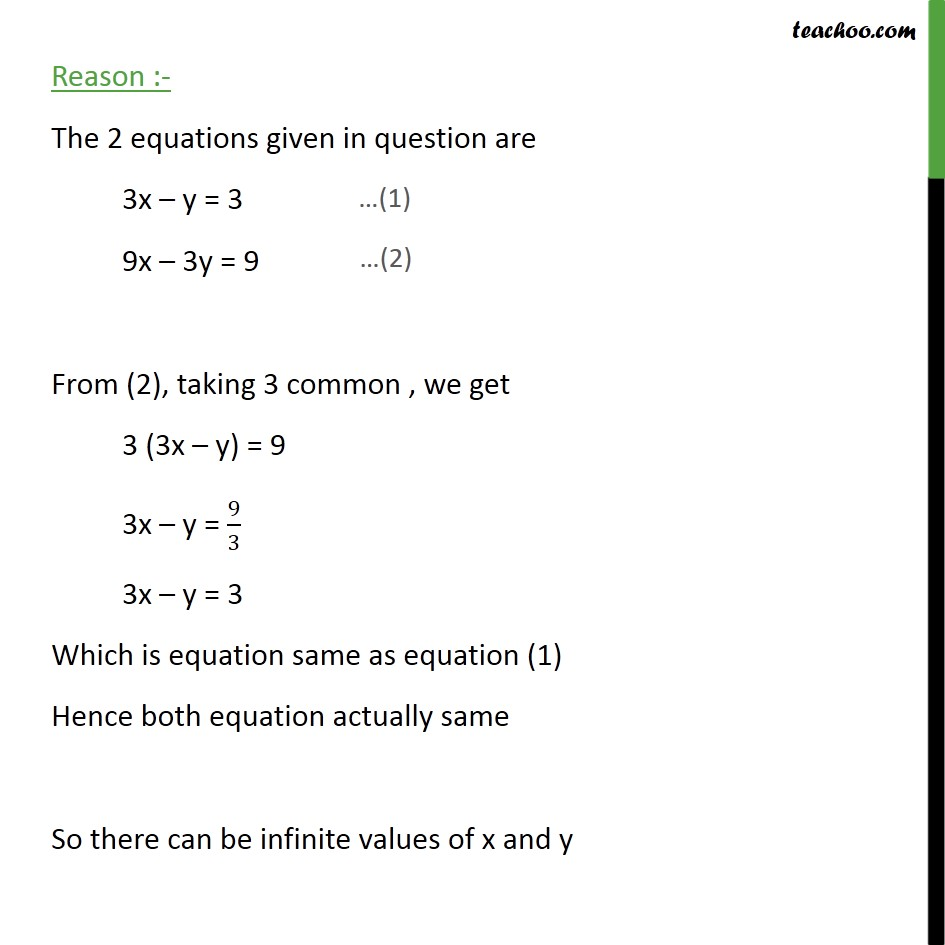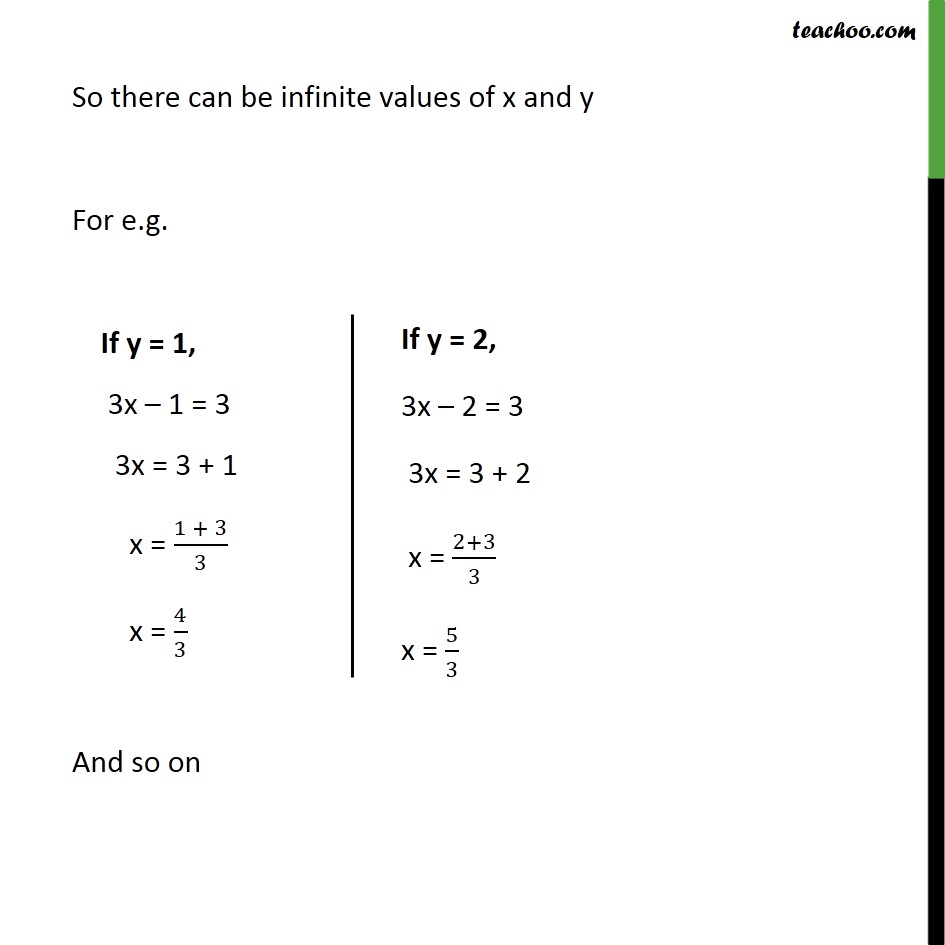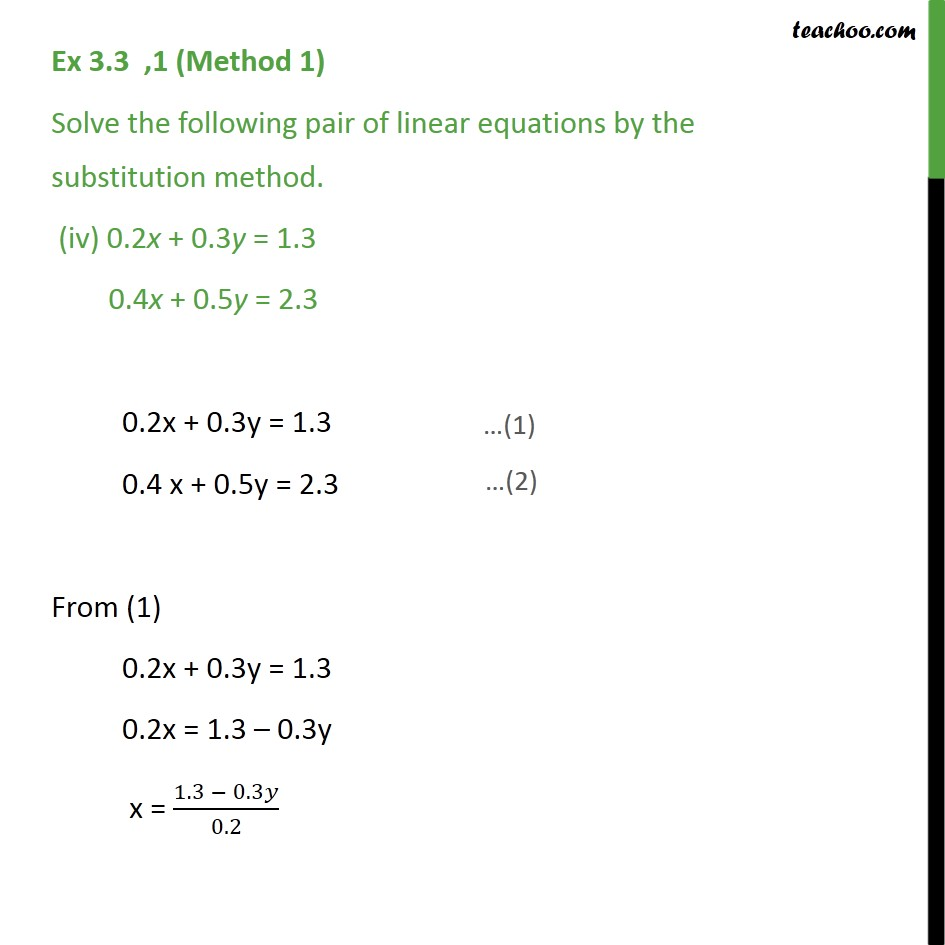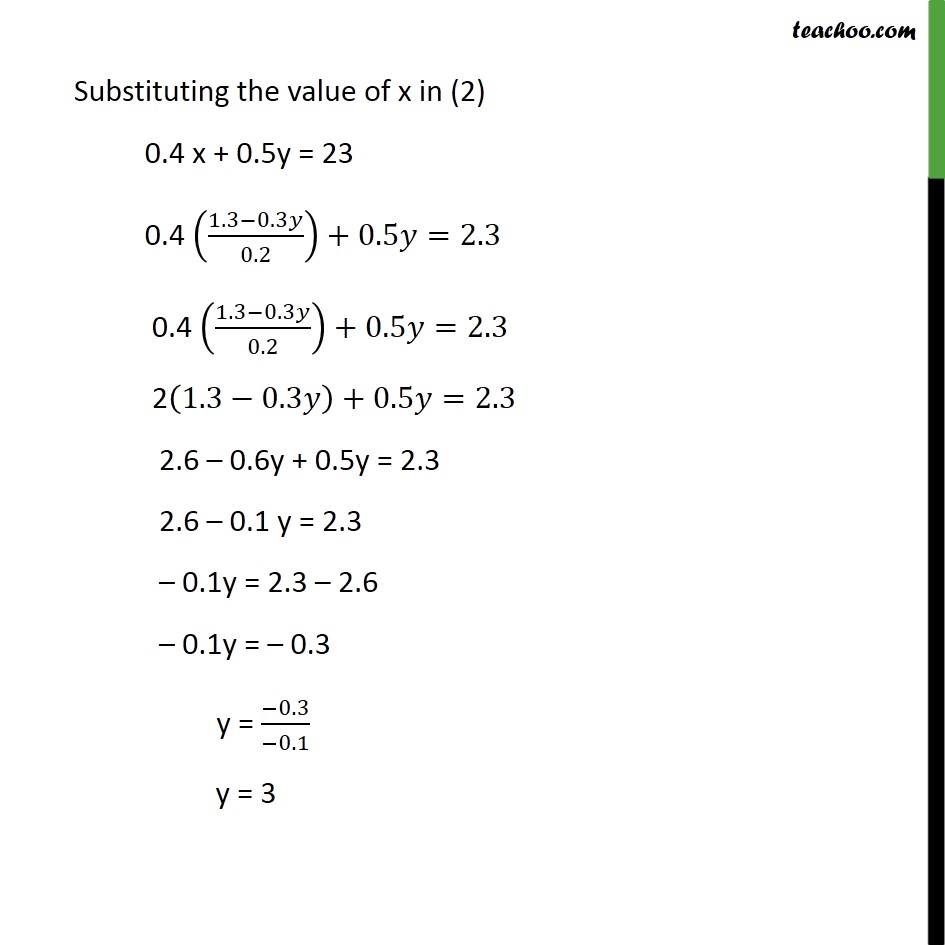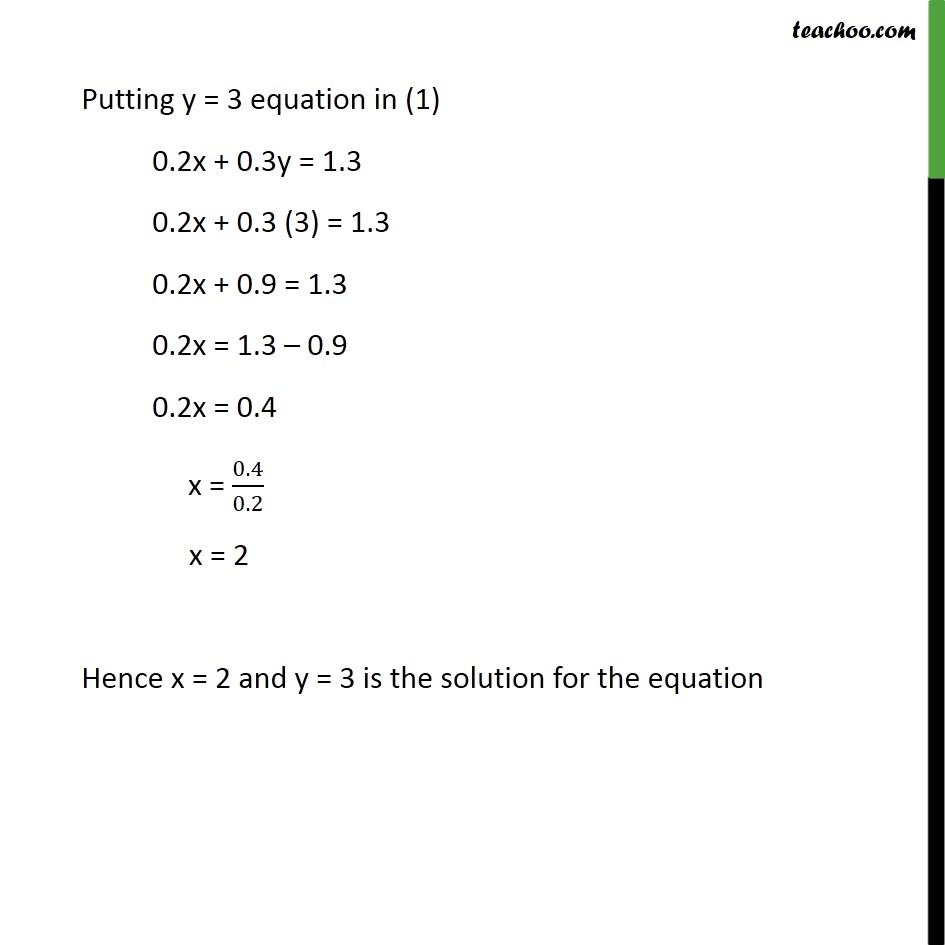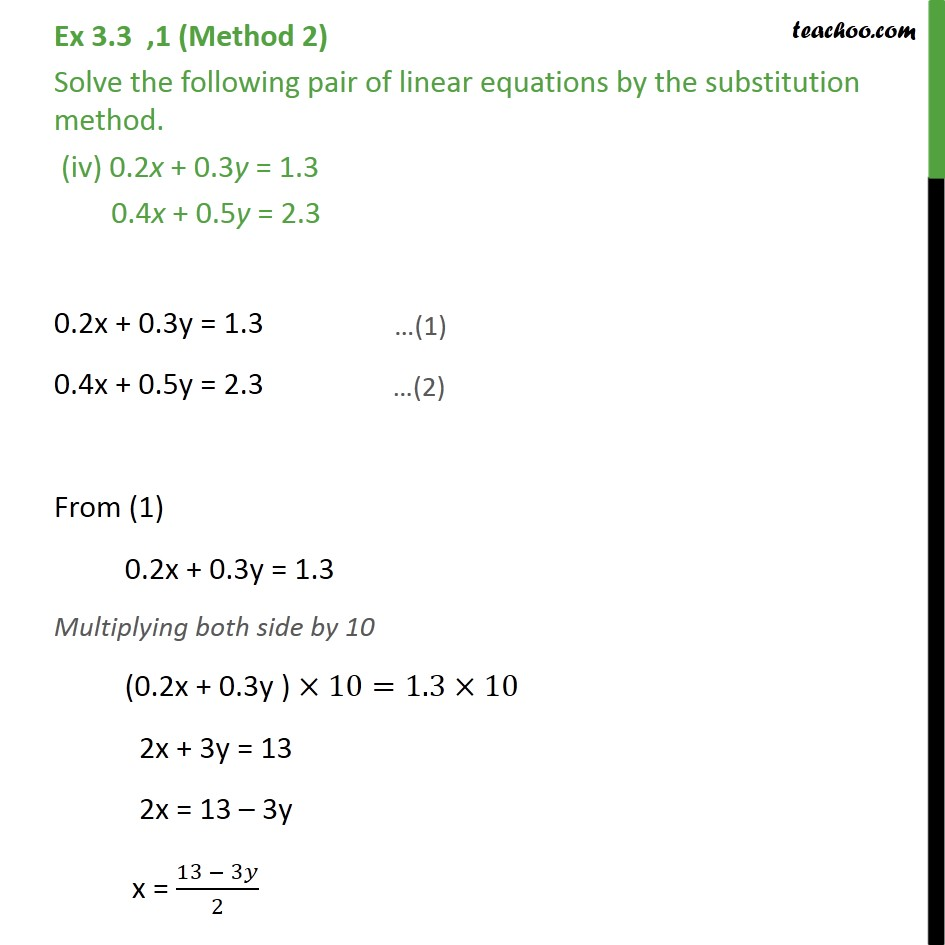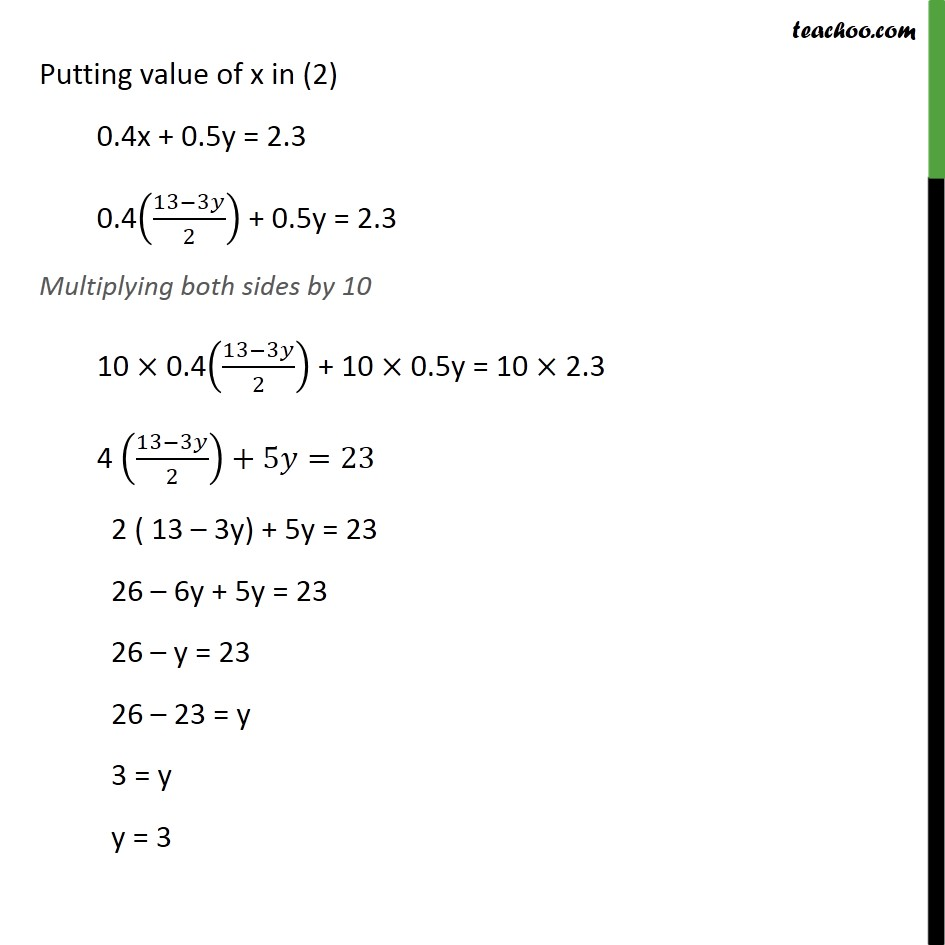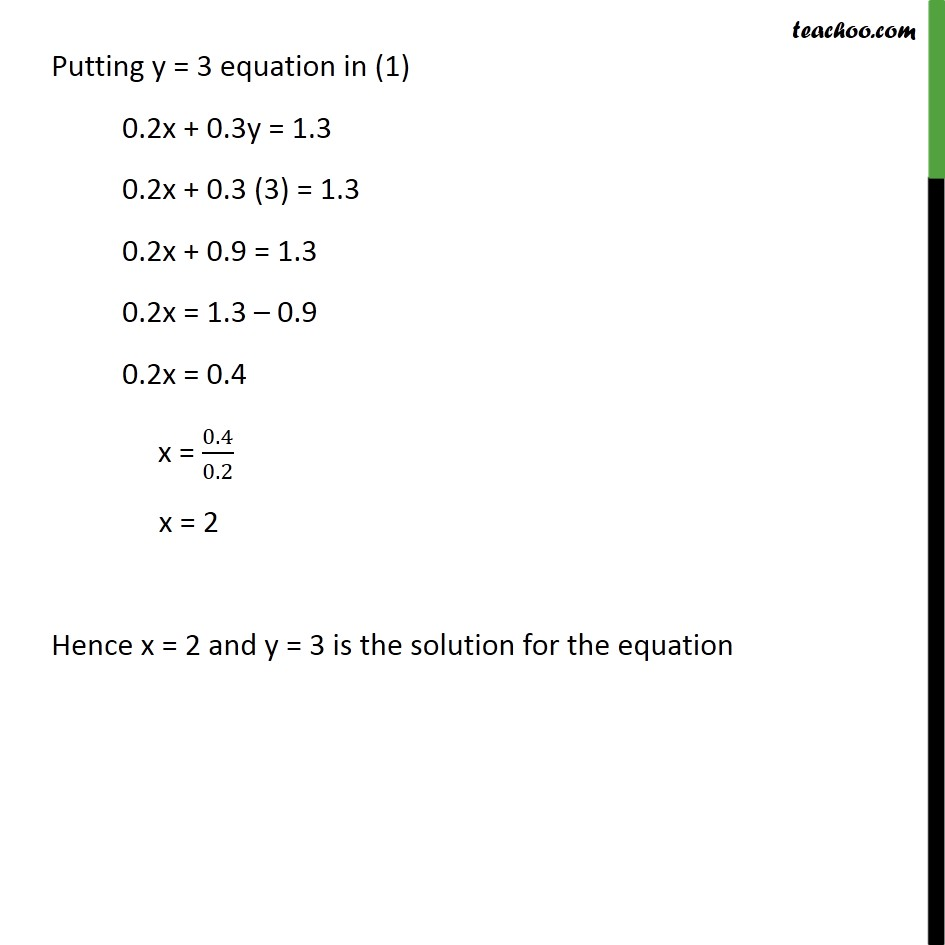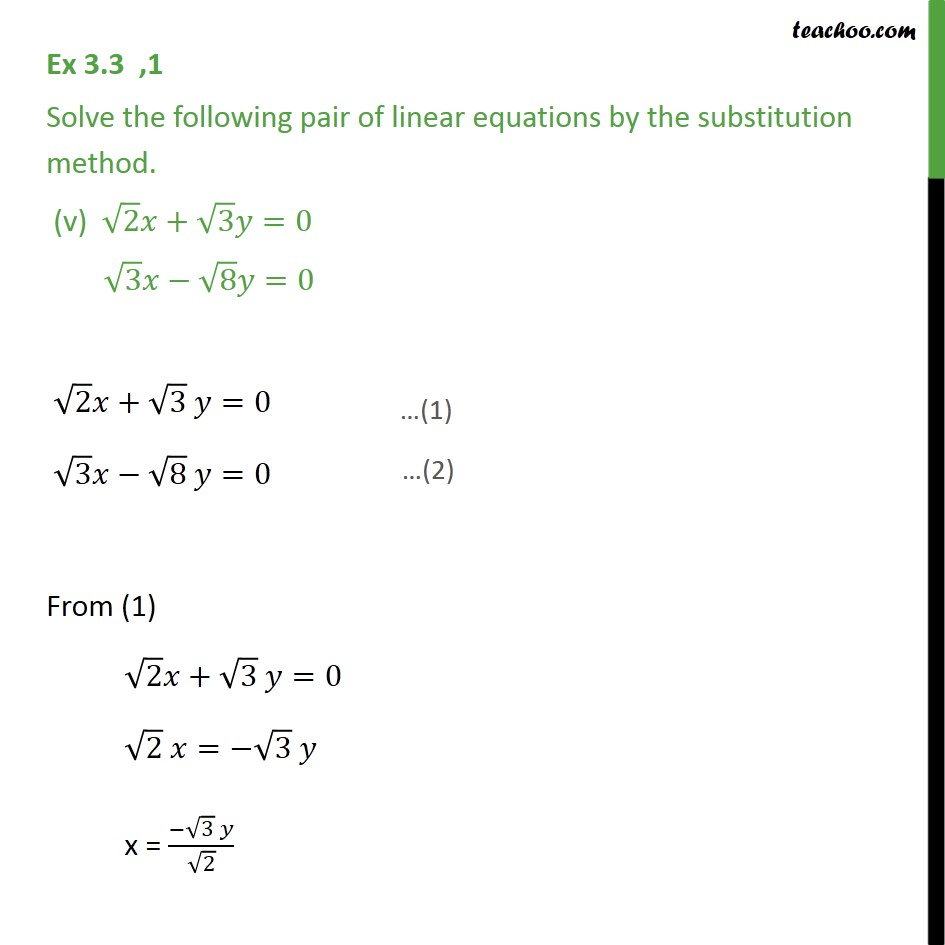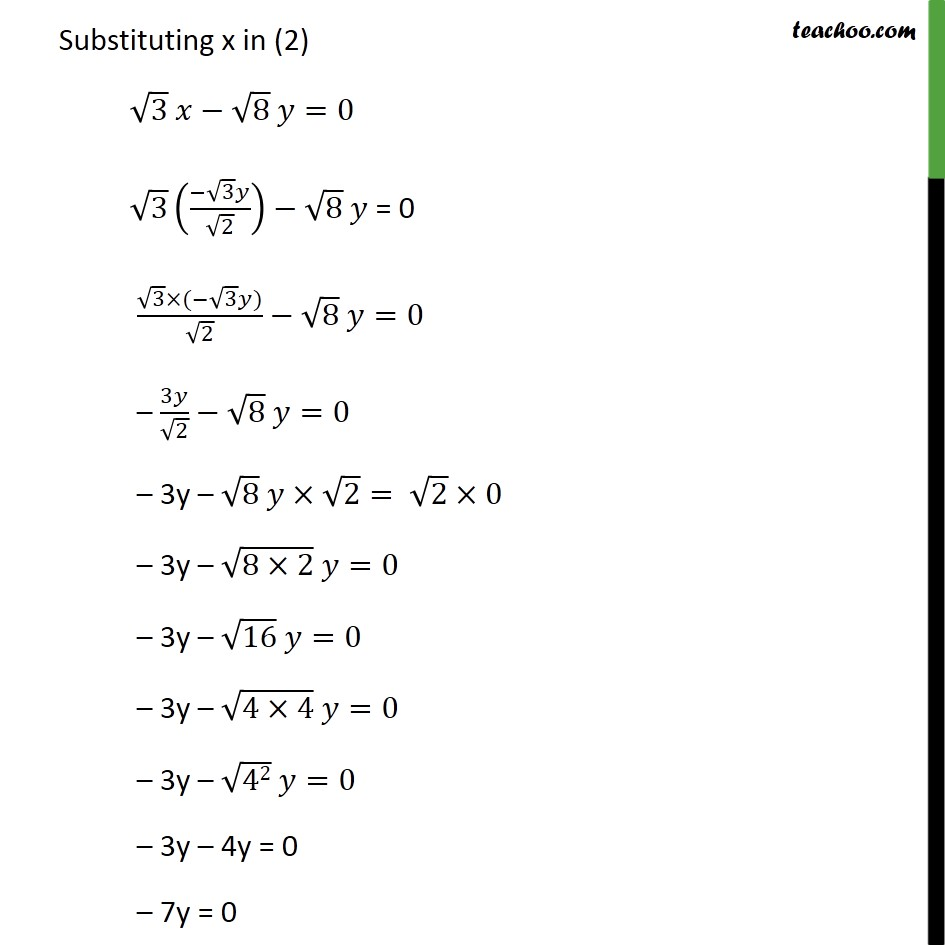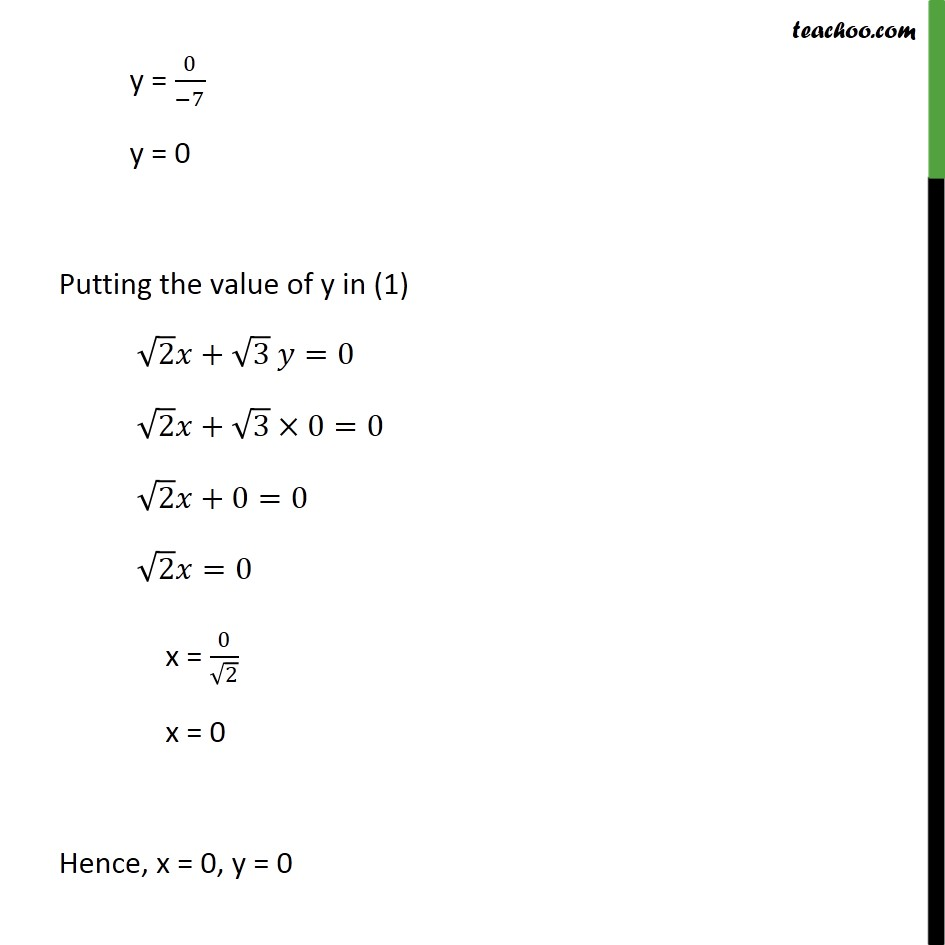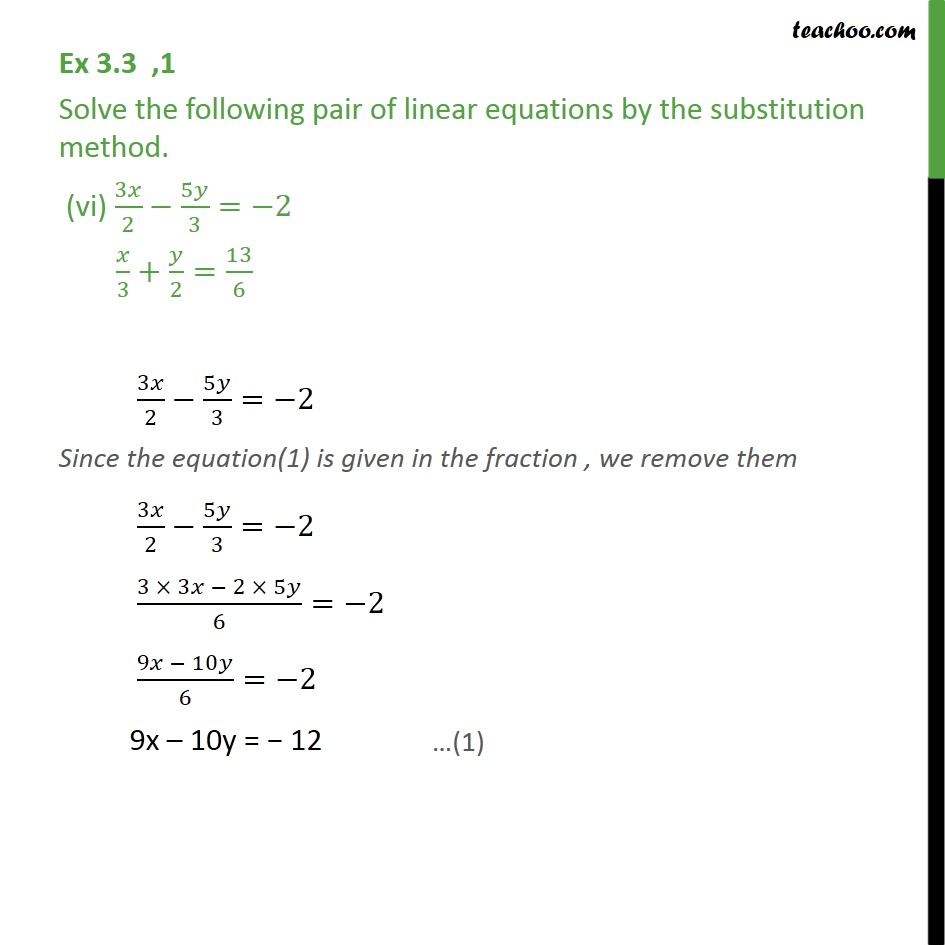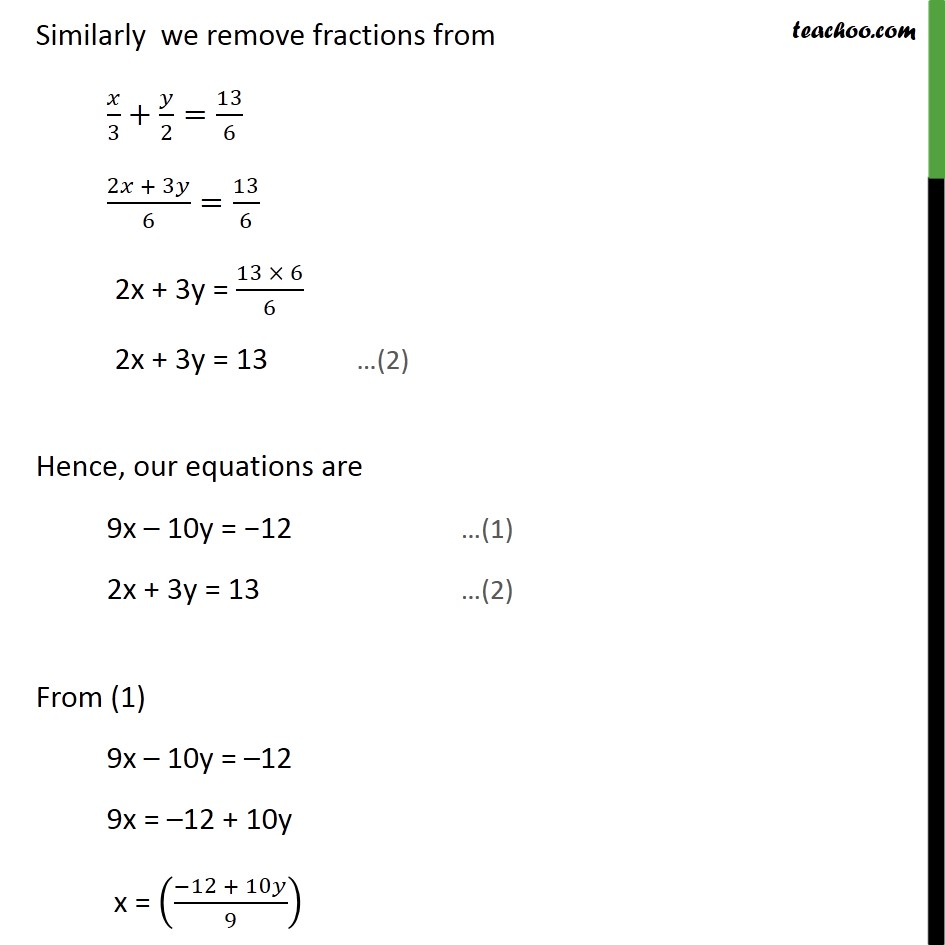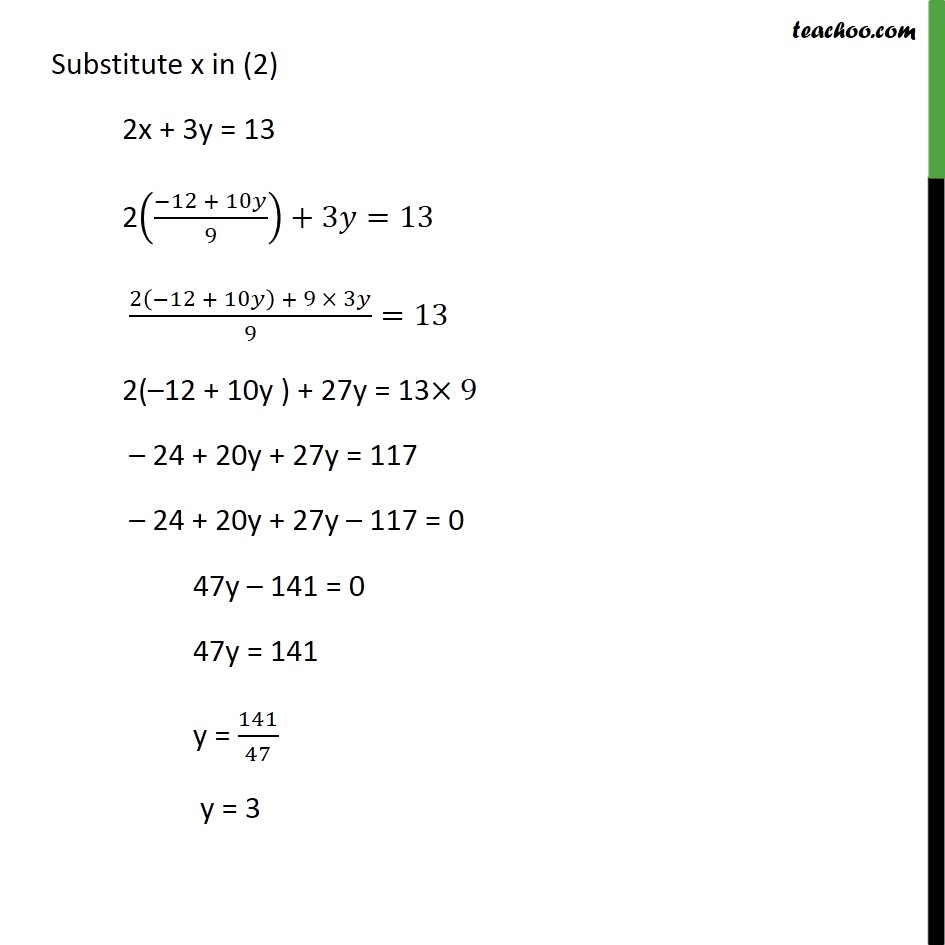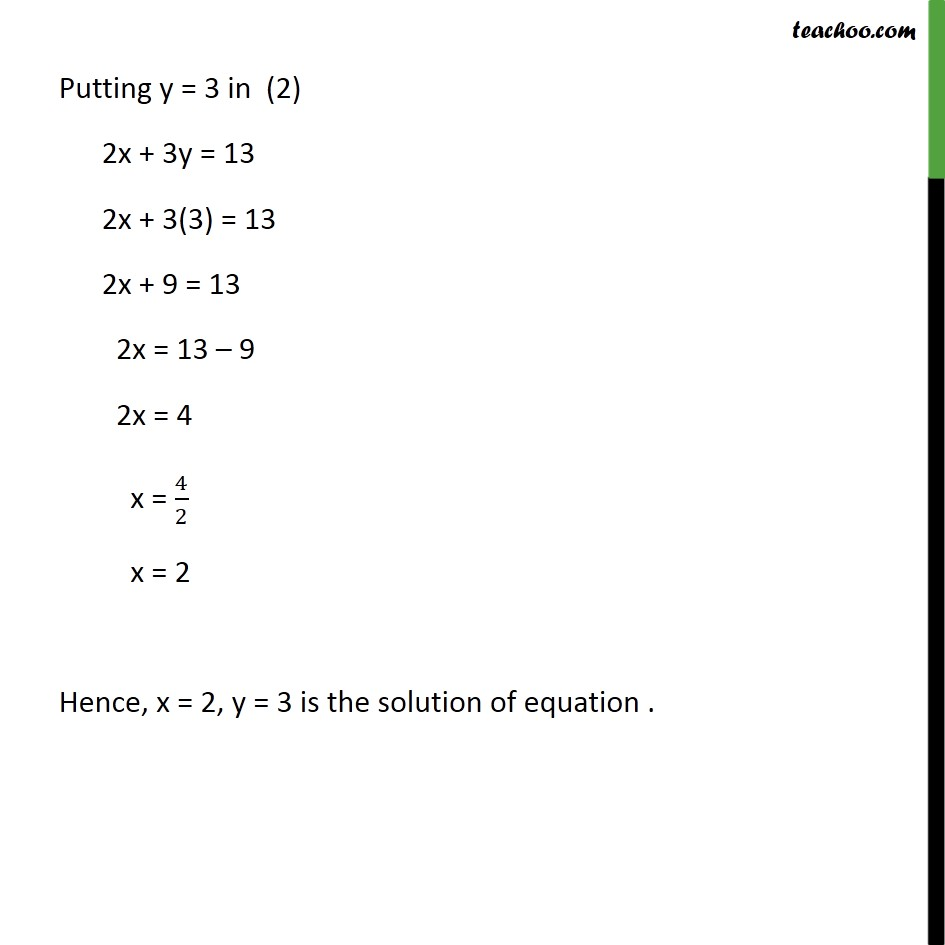1. Chapter 3 Class 10 Pair of Linear Equations in Two Variables
2. Serial order wise
3. Ex 3.3

Transcript

Ex 3.3 ,1 Solve the following pair of linear equations by the substitution method. (i) x + y = 14 x y = 4 x + y = 14 x y = 4 From equation (1) x + y = 14 x = 14 y Substituting x = 14 y in equation (2) x y = 4 (14 y) y = 4 14 y y = 4 14 2y = 4 2y = 4 14 2y = 10 y = ( 10)/( 2) y = 5 Putting y = 5 in (2) x y = 4 x = y + 4 x = 5 + 4 x = 9 Hence, x = 9, y = 5 is the solution of the above equation Ex 3.3 ,1 Solve the following pair of linear equations by the substitution method. (ii) s t = 3 /3+ /2=6 s t = 3 /3+ /2=6 From (1) s t = 3 s = 3 + t Substituting s = 3 + t in (2) (3 + )/3+ /2=6 ((3 + ) 2 + 3)/(3 2)=6 (6 + 2 + 3 )/6=6 (6 + 5 )/6=6 6 + 5t = 6 6 6 + 5t = 36 5t = 36 6 5t = 30 t = 30/5 t = 6 Putting t = 6 in (1) s t = 3 s = 3 + 6 s = 9 Hence, s = 9, t = 6 Ex 3.3 ,1 Solve the following pair of linear equations by the substitution method. (iii) 3x y = 3 9x 3y = 9 3x y = 3 9x 3y = 9 Solving (1) 3x y = 3 3x = y + 3 x = ( + 3)/3 Putting value of x in (2) 9x 3y = 9 9(( + 3)/3) 3 =9 3(y + 3) 3y = 9 3y + 9 3y = 9 3y 3y + 9 = 9 0 + 9 = 9 9 = 9 The statement is true for all values of x Hence, there is no single value of x as the answer for this question Reason :- The 2 equations given in question are 3x y = 3 9x 3y = 9 From (2), taking 3 common , we get 3 (3x y) = 9 3x y = 9/3 3x y = 3 Which is equation same as equation (1) Hence both equation actually same So there can be infinite values of x and y So there can be infinite values of x and y For e.g. If y = 1, 3x 1 = 3 3x = 3 + 1 x = (1 + 3)/3 x = 4/3 And so on Ex 3.3 ,1 (Method 1) Solve the following pair of linear equations by the substitution method. (iv) 0.2x + 0.3y = 1.3 0.4x + 0.5y = 2.3 0.2x + 0.3y = 1.3 0.4 x + 0.5y = 2.3 From (1) 0.2x + 0.3y = 1.3 0.2x = 1.3 0.3y x = (1.3 0.3 )/0.2 Substituting the value of x in (2) 0.4 x + 0.5y = 23 0.4 ((1.3 0.3 )/0.2)+0.5 =2.3 0.4 ((1.3 0.3 )/0.2)+0.5 =2.3 2(1.3 0.3 )+0.5 =2.3 2.6 0.6y + 0.5y = 2.3 2.6 0.1 y = 2.3 0.1y = 2.3 2.6 0.1y = 0.3 y = ( 0.3)/( 0.1) y = 3 Putting y = 3 equation in (1) 0.2x + 0.3y = 1.3 0.2x + 0.3 (3) = 1.3 0.2x + 0.9 = 1.3 0.2x = 1.3 0.9 0.2x = 0.4 x = 0.4/0.2 x = 2 Hence x = 2 and y = 3 is the solution for the equation Ex 3.3 ,1 (Method 2) Solve the following pair of linear equations by the substitution method. (iv) 0.2x + 0.3y = 1.3 0.4x + 0.5y = 2.3 0.2x + 0.3y = 1.3 0.4x + 0.5y = 2.3 From (1) 0.2x + 0.3y = 1.3 Multiplying both side by 10 (0.2x + 0.3y ) 10=1.3 10 2x + 3y = 13 2x = 13 3y x = (13 3 )/2 Putting value of x in (2) 0.4x + 0.5y = 2.3 0.4((13 3 )/2) + 0.5y = 2.3 Multiplying both sides by 10 10 0.4((13 3 )/2) + 10 0.5y = 10 2.3 4 ((13 3 )/2)+5 =23 2 ( 13 3y) + 5y = 23 26 6y + 5y = 23 26 y = 23 26 23 = y 3 = y y = 3 Putting y = 3 equation in (1) 0.2x + 0.3y = 1.3 0.2x + 0.3 (3) = 1.3 0.2x + 0.9 = 1.3 0.2x = 1.3 0.9 0.2x = 0.4 x = 0.4/0.2 x = 2 Hence x = 2 and y = 3 is the solution for the equation Ex 3.3 ,1 Solve the following pair of linear equations by the substitution method. (v) 2 + 3 =0 3 8 =0 2 + 3 =0 3 8 =0 From (1) 2 + 3 =0 2 = 3 x = ( 3 )/ 2 Substituting x in (2) 3 8 =0 3 (( 3 )/ 2) 8 = 0 ( 3 ( 3 ))/ 2 8 =0 3 / 2 8 =0 3y 8 2= 2 0 3y (8 2) =0 3y 16 =0 3y (4 4) =0 3y 42 =0 3y 4y = 0 7y = 0 y = 0/( 7) y = 0 Putting the value of y in (1) 2 + 3 =0 2 + 3 0=0 2 +0=0 2 =0 x = 0/ 2 x = 0 Hence, x = 0, y = 0 Ex 3.3 ,1 Solve the following pair of linear equations by the substitution method. (vi) 3 /2 5 /3= 2 /3+ /2=13/6 3 /2 5 /3= 2 Since the equation(1) is given in the fraction , we remove them 3 /2 5 /3= 2 (3 3 2 5 )/6= 2 (9 10 )/6= 2 9x 10y = 12 Similarly we remove fractions from /3+ /2=13/6 (2 + 3 )/6=13/6 2x + 3y = (13 6)/6 2x + 3y = 13 Hence, our equations are 9x 10y = 12 (1) 2x + 3y = 13 (2) From (1) 9x 10y = 12 9x = 12 + 10y x = (( 12 + 10 )/9) Substitute x in (2) 2x + 3y = 13 2(( 12 + 10 )/9)+3 =13 (2( 12 + 10 ) + 9 3 )/9=13 2( 12 + 10y ) + 27y = 13 9 24 + 20y + 27y = 117 24 + 20y + 27y 117 = 0 47y 141 = 0 47y = 141 y = 141/47 y = 3 Putting y = 3 in (2) 2x + 3y = 13 2x + 3(3) = 13 2x + 9 = 13 2x = 13 9 2x = 4 x = 4/2 x = 2 Hence, x = 2, y = 3 is the solution of equation .

Ex 3.3